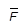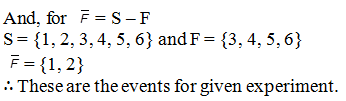• +91 9971497814
• info@interviewmaterial.com

# RD Chapter 33- Probability Ex-33.2 Interview Questions Answers

### Related Subjects

Question 1 : A coin is tossed. Find the total number of elementary events and also the total number of events associated with the random experiment.

Given: A coin is tossed.
When a coin is tossed, there will be two possible outcomes, Head (H) and Tail (T).
Since, the no. of elementary events is 2 {H, T}
We know, if there are n elements in a set, then the number of total element in its subset is 2n.
So, the total number of the experiment is 4,
There are 4 subset of S = {H}, {T}, {H, T} and Փ
∴ There are total 4 events in a given experiment.

Question 2 : List all events associated with the random experiment of tossing of two coins. How many of them are elementary events?

Given: Two coins are tossed once.
We know, when two coins are tossed then the no. of possible outcomes are 22 = 4
So, the Sample spaces are {HH, HT, TT, TH}
∴ There are total 4 events associated with the given experiment.

Question 3 :
Three coins are tossed once. Describe the following events associated with this random experiment:
A = Getting three heads, B = Getting two heads and one tail, C = Getting three tails, D = Getting a head on the first coin.
(i) Which pairs of events are mutually exclusive?
(ii) Which events are elementary events?
(iii) Which events are compound events?

Given: There are three coins tossed once.
When three coins are tossed, then the sample spaces are:
S = {HHH, HHT, HTH, HTT, THH, THT, TTH, TTT}
So, according to the question,
A = {HHH}
B = {HHT, HTH, THH}
C = {TTT}
D = {HHH, HHT, HTH, HTT}
Now, A⋂ B = Փ,
A ⋂ C = Փ,
A ⋂ D = {HHH}
B⋂ C = Փ,
B ⋂ D = {HHT, HTH}
C ⋂ D = Փ
We know that, if the intersection of two sets are null or empty it means both the sets are Mutually Exclusive.
(i) Events A and B, Events A and C, Events B and C and events C and D are mutually exclusive.
(ii) Here, We know, if an event has only one sample point of a sample space, then it is called elementary events.
So, A and C are elementary events.
(iii) If there is an event that has more than one sample point of a sample space, it is called a compound event.
Since, B ⋂ D = {HHT, HTH}
So, B and D are compound events.

Question 4 :
In a single throw of a die describe the following events:
(i) A = Getting a number less than 7
(ii) B = Getting a number greater than 7
(iii) C = Getting a multiple of 3
(iv) D = Getting a number less than 4
(v) E = Getting an even number greater than 4.
(vi) F = Getting a number not less than 3.
Also, find A ∪ B, A ∩ B, B ∩ C, E ∩ F, D ∩ F and .

Given: A dice isthrown once.

Let us find the givenevents, and also find A  B,A ∩ B, B ∩ C, E ∩ F, D ∩ F andS = {1, 2, 3, 4, 5, 6}

According to thesubparts of the question, we have certain events as:

(i) A = getting anumber below 7

So, the sample spacesfor A are:

A = {1, 2, 3, 4, 5, 6}

(ii) B = Getting anumber greater than 7

So, the sample spacesfor B are:

B = {Փ}

(iii) C = Gettingmultiple of 3

So, the Sample spaceof C is

C = {3, 6}

(iv) D = Getting anumber less than 4

So, the sample spacefor D is

D = {1, 2, 3}

(v) E = Getting aneven number greater than 4.

So, the sample spacefor E is

E = {6}

(vi) F = Getting anumber not less than 3.

So, the sample spacefor F is

F = {3, 4, 5, 6}

Now,

A = {1, 2, 3, 4, 5, 6}and B = {Փ}

A B = {1, 2, 3, 4, 5, 6}

A = {1, 2, 3, 4, 5, 6}and B = {Փ}

A B = {Փ}

B = {Փ} and C = {3, 6}

B C = {Փ}

F = {3, 4, 5, 6} and E= {6}

E F = {6}

E = {6} and D = {1, 2,3}

D F = {3}Question 5 :
Three coins are tossed. Describe
(i) two events A and B which are mutually exclusive.
(ii) three events A, B and C which are mutually exclusive and exhaustive.
(iii) two events A and B which are not mutually exclusive.
(iv) two events A and B which are mutually exclusive but not exhaustive.

Given: Three coins aretossed.

When three coins aretossed, then the sample space is

S = {HHH, HHT, HTH,HTT, THH, THT, TTH, TTT}

Now, the subparts are:

(i) The two eventswhich are mutually exclusive are when,

A: getting no tails

Then, A = {HHH} and B= {TTT}

So, the intersectionof this set will be null. Or, the sets are disjoint.

(ii) Three eventswhich are mutually exclusive and exhaustive are:

So, A = {TTT} B ={TTH, THT, HTT} and C = {HHH, HHT, HTH, THH}

Since, A B = B C = C A = Փ and

A B C = S

(iii) The two eventsthat are not mutually exclusive are:

So, A = {HHH} B ={HHH, HHT, HTH, THH}

Hence, A B = {HHH} = Փ

(iv) The two eventswhich are mutually exclusive but not exhaustive are:

B: getting exactly onetail

So, A = {HTT, THT,TTH} and B = {HHT, HTH, THH}

It is because A B = Փ but A B ≠ S

Question 6 :
A die is thrown twice. Each time the number appearing on it is recorded. Describe the following events:
(i) A = Both numbers are odd.
(ii) B = Both numbers are even
(iii) C = sum of the numbers is less than 6.
Also, find A ∪ B, A ∩ B, A ∪ C, A ∩ C. Which pairs of events are mutually exclusive?

Given: A dice is thrown twice. And each time number appearing on it is recorded.
When the dice is thrown twice then the number of sample spaces are 62 = 36
Now,
The possibility both odd numbers are:
A = {(1, 1), (1, 3), (1, 5), (3, 1), (3, 3), (3, 5), (5, 1), (5, 3), (5, 5)}
Since, possibility of both even numbers is:
B = {(2, 2), (2, 4), (2, 6), (4, 2), (4, 4), (4, 6), (6, 2), (6, 4), (6, 6)}
And, possible outcome of sum of the numbers is less than 6.
C = {(1, 1)(1, 2)(1, 3)(1, 4)(2, 1)(2, 2)(2, 3)(3, 1)(3, 2)(4, 1)}
Hence,
(AՍB) = {(1, 1), (1, 3), (1, 5), (3, 1), (3, 3), (3, 5), (5, 1), (5, 3), (5, 5) (2, 2)(2, 4)(2, 6)(4, 2)(4, 4)(4, 6)(6, 2)(6, 4)(6, 6)}
(AՌB) = {Փ}
(AUC) = {(1, 1), (1, 3), (1, 5), (3, 1), (3, 3), (3, 5), (5, 1), (5, 3), (5, 5) (1, 2)(1, 4)(2, 1)(2, 2)(2, 3)(3, 1)(3, 2)(4, 1)}
(AՌC) = {(1, 1), (1, 3), (3, 1)}
∴ (AՌB) = Փ and (AՌC) ≠ Փ, A and B are mutually exclusive, but A and C are not.

Todays Deals### RD Chapter 33- Probability Ex-33.2 Contributorskrishan

Name:
Email:

# Latest News# 9000 interview questions in different categories